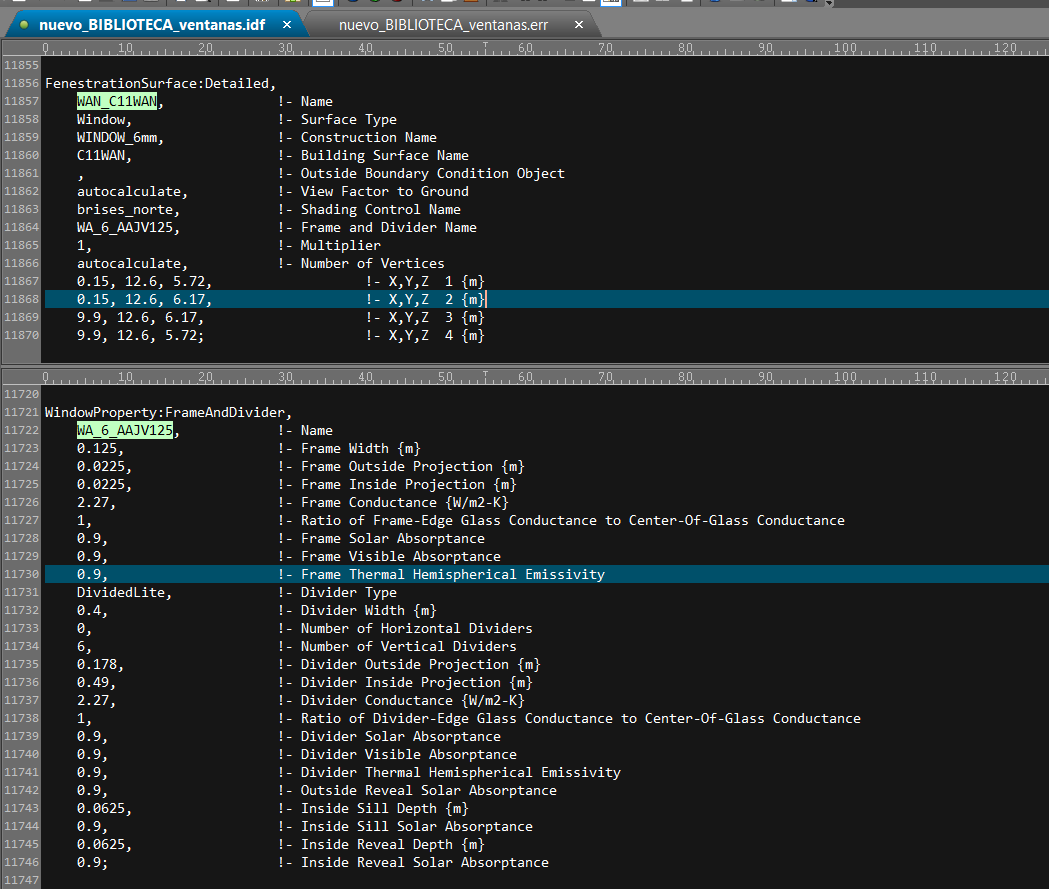Question-and-Answer Resource for the Building Energy Modeling Community
Get started with the Help page

Error: ** Severe ** ProcessSurfaceVertices: Divider area exceeds glazed opening for window...During the simulation several errors of this type occur: "The area of the divider exceeds the glass opening for the window". Here is an example using one of the windows:ERROR:

* Severe * ProcessSurfaceVertices: Divider area exceeds window opening for window WAN_C11WAN * ~~~ * Window surface = [4.38] m2, dividing area = [23.40] m2.

PROOF:

The area of the window is: 9.75 x 0.45 = 4.38 .... Ok The area of the dividers: 0.4 x 0.45 x 6 = 1.08 (the vertical dividers are those parallel to the sides link text

To get the 23.4 m2 that the program gives, I must multiply the width of the divider for the width of the window instead of the height, that sounds wrong to me...

Area according to the program: 0.4 x 9.75 x 6 = 23.4 ...

So, I must enter the vertical dividers (parallels to the sides) like horizontal dividers (parallels to the top)? These is a program mistake or I am wrong??

edit retag close merge delete

Divider area is calculated as follows.

DivArea = DivWidth * ( HorDividers * Surface.Width + VertDividers * Surface.Height - HorDividers * VertDividers * DivWidth );
DividerArea = DivArea * Surface.Multiplier;

Since Surface.Multiplier = 1, for HorDividers=0, VertDividers = 6, DivArea = 1.08 (as you correctly calculated). and for HorDividers=6, VertDividers = 0, DivArea = 23.4. (seems incorrect and is what E+ is calculating) Debugging idf with E+ source code or posting link to actual idf will help in seeing what is going on inside E+.

Sort by » oldest newest most voted

From window coordinates, your window seems to be horizontal one. So it is not clear to me what "horizontal" and "vertical" divider means in this case. Perhaps they are reversed. Try specifying 0 vertical dividers and 6 horizontal dividers.

more